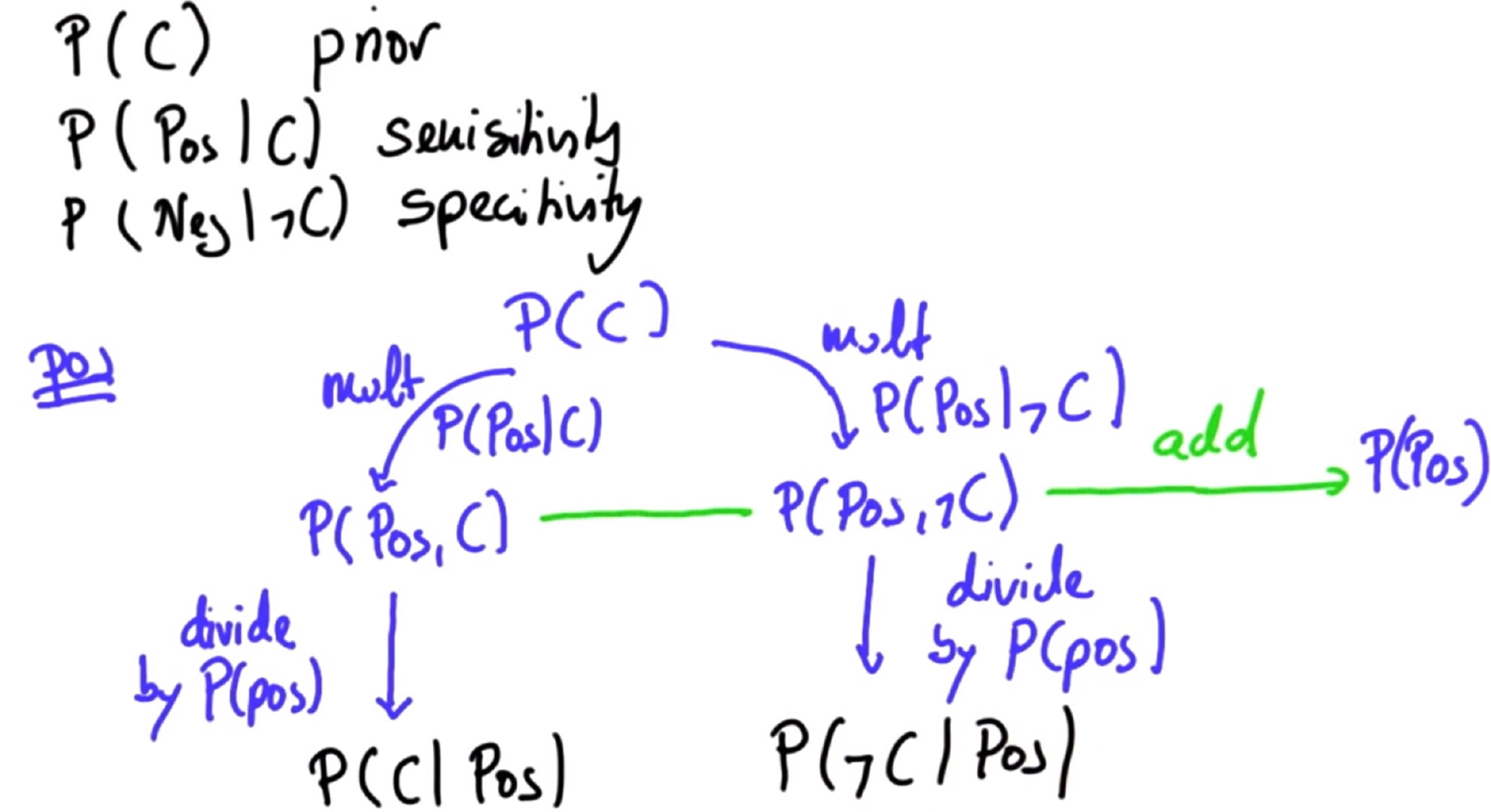# 无人技术入门——贝叶斯定理

## June 9, 2021 • 无人技术### 关于符号

P(A|B)P(A|B) 等等，其中一个字母代表假设，另一个代表观察结果。符号不同，但概念相同。只要你对概念熟悉，你就能从容应对不同的符号！

# 1.先验概率

• 确定P(H)
• 得到P(H)后，即可推导出P(¬H)

# 2.条件/测试概率

• 确定P(T|H)和P(T|¬H)
• 得到P(T|H)后，即可推导出P(¬T|H)

# 3.联合概率

• P(H,T)=P(T|H)⋅P(H)
• 类似地,P(¬H,T)=P(T|¬H)⋅P(¬H)

# 4.总概率

• P(T)=P(H,T)+P(¬H,T)

# 4.后验概率（最后一步）

• P(H|T)= P(T|H)⋅P(H)/P(T)

# 问题：

• 在所有人口中，有1%得了癌症——P(C)=0.1
• 90%的癌症患者在接受癌症检测的血液检测结果为阳性，这意味着90%的可能性检测出癌症——P(Pos|C)=0.9
• 5%的人会有误报，也就是说有5%的可能性，会出现该检测在人们没有癌症的时候呈现阳性结果的情况——P(Pos|¬C)=0.05